## Octal Number System (Definition, Conversions & Examples):

The number system with base (or radix) eight is known as the octal number system. Although we can use any eight digits, it is customary to use the first eight decimal digits: 0, 1, 2, 3, 4, 5, 6, 7. There is no 8 or 9 in octal number code. These digits 0 through 7, have exactly the same physical meaning as in decimal number system. The digit positions in octal number system have weights as follows.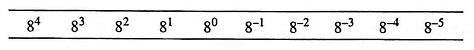In digital systems, binary numbers are required to be entered and certain results or status signals are required to be displayed. It is highly inconvenient to handle long strings of binary numbers. It may cause errors also. Therefore, octal numbers are used for entering the binary data and displaying certain information. The octal number system requires one-third in length as compared to binary numbers. Thus from the users point of view, it would be comparatively much easier to handle the input and output data of a digital computer in octal form. Moreover, the printouts are more compact and easy to read. Conversion from binary-to-octal and octal-to-binary is also quick and simple. In fact, octal number system are used to represent binary numbers because of ease of conversion and compactness. Since digital circuits can process only zeros and ones, the octal numbers have to be converted into binary form employing special circuits known as octal-to-binary converters before being processed by the digital circuits.

### Octal-To-Decimal Conversion:

An octal number system can be easily converted to its decimal equivalent by multiplying each octal digit by its positional weight.

For example,

3148 = 3 x (82) + 1 (81) + 4 (80) = 192 + 8 + 4 = 20410

Another example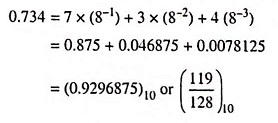Example 44.30: Convert octal 756 to decimal.

Solution: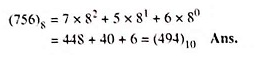Example 44.31: Convert the following number (36.125)8 = ( )10

Solution:### Decimal-To-Octal Conversion:

A decimal integer can be converted to octal by using the same repeated division method, called the double dabble method, that we used in the decimal-to-binary conversion, but with a division factor of 8 rather than 2. The following examples will illustrate the procedure.

Example 44.32: Convert the following: (540)10 = ( )8

Solution: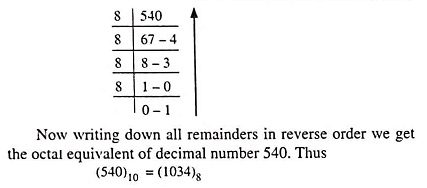Example 44.33: Convert (0.45)10 = ( )8.

Solution: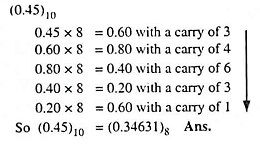### Octal-To-Binary Conversion:

Because 8 (the base of octal numbers) is the third power of 2 (the base of binary number), the conversion from octal to binary can be performed by converting each octal digit to its 3-bit binary equivalent. The eight possible digits are converted as indicated below in Table 44.2.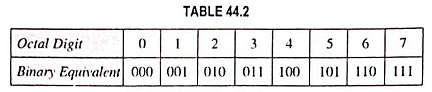Using these conversions, any octal number system can be converted to binary by individually converting each digit. The following examples will illustrate the procedure.

Example 44.36: Convert the following number (375.37)8 = ( )2

Solution: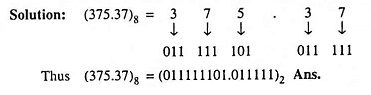### Binary-To-Octal Conversion:

Conversion of a binary number to an octal number is simply the reverse of the foregoing process. The bits of the binary number are grouped into groups of three bits starting from LSB towards MSB for integer part and then each group of three bits is replaced by its octal equivalent. Zeros are added, as required to complete a 3-bit group. For the fractional part, the above procedure is repeated from the bit next to the binary point. The following examples will illustrate the procedure.

Example 44.37: Convert (111000)2 to octal.

Solution: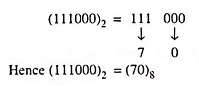Example 44.38: Do the following conversion: (101010011.1101)2 to ( )8

Solution: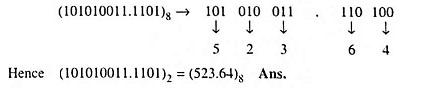### Octal Arithmetic:

Normally octal arithmetic operations using octal representation of numbers are not performed. This number system is normally employed to enter long strings of binary data into a digital system like a microcomputer. This makes the task of the operator easier. Arithmetic operations can be performed by converting the octal numbers into binary numbers and then using the rules of binary arithmetic. Following few examples are considered for illustration.

Scroll to Top